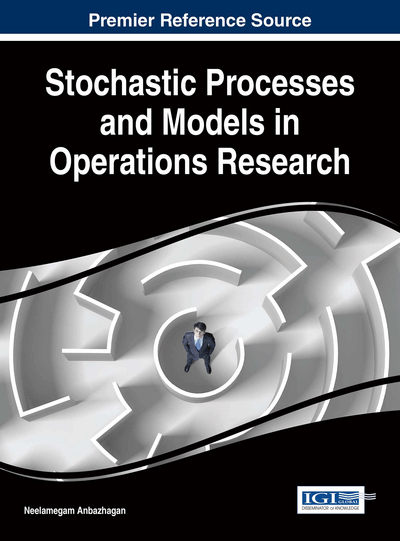# Building Fuzzy Models of Stochastic Processes to Determine Probabilistic and Linguistic Types of Uncertainty

Anna Walaszek-Babiszewska (Opole University of Technology, Poland)
DOI: 10.4018/978-1-5225-0044-5.ch014

## Abstract

In the chapter an advanced fuzzy modeling methods have been presented which joints fuzzy and probabilistic approaches. The Zadeh's notions of probability of imprecise events has served as a basis for determining probability distributions of linguistic random variable, stochastic process with fuzzy states, and fuzzy-valued stochastic Markov process. Rule based fuzzy representations have been also presented. Exemplary calculations illustrate the way of building fuzzy models, using empirical data or theoretical probability distribution.
Chapter Preview
Top

## Basic Definitions

### Fuzzy Set

The notion of a fuzzy set is a generalization of a classic set, defined in mathematics by a characteristic function,, which assigns number 1 to every element x from X, if x belongs to A, and 0 if x does not belong to A.

Fuzzy set A, as a subset of a certain space of consideration X, is determined by a membership function,, which assigns a real number in the interval [0,1] to every element,. The values of functionrepresent membership degrees of particular elements x in A (Zadeh, 1965).

Fuzzy set A in a space of consideration,, is denoted by a set of pairs

(1) where the membership function can be given in some analytic form, e.g.

(2)

When the space of consideration is a finite set,, fuzzy set A is denoted as a sum of elements with the membership degrees, in the following form

. (3)

## Complete Chapter List

Search this Book:
Reset